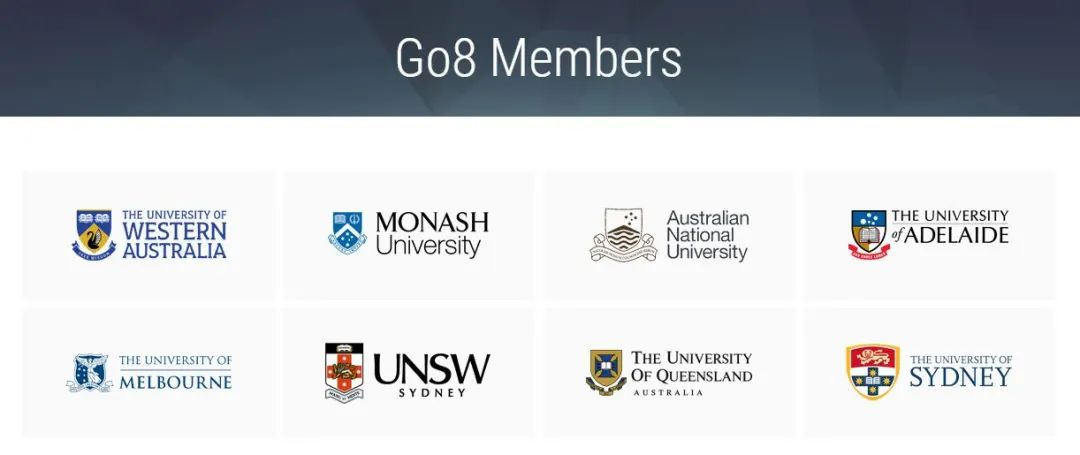# 澳大利亚八大名校A-Level申请要求汇总

Go8的成员包括：用A-level成绩申请澳洲大学适用于以下计算方法：首先A-Level分以下六个等级，A. B. C. D. E. U。A=5，B=4，C=3，D=2，E=1，U=fail。取最好的三门分数。

A-Level成绩换算：
A*=6
A=5
B=4
C=3
D=2
E=1

A-Level成绩要求：10，人文/IT/科学12，商科13，会计14，工程/国际关系15，精算16,17,18

IELTS成绩要求：总分6.5且每一项不低于6.0

A-Level成绩换算：
A*=140
A=120
B=100
C=80
D=60
E=40
a=60
b=50
c=40
d=30
e=20

A-Level成绩要求：建筑/传媒/商/法律AAA，IT/经济/工程AAB/ABC，药剂/护理ABB，计算机/人文/科学/环境/教育ABC/BBB

IELTS成绩要求：总分6.5-7.5，每门不得低于6.0(不同专业不同要求，其中商学院所有专业都要求7分以上)

A-Level成绩换算：
A*=6
A=5
B=4
C=3
D=2
E=1

A-Level成绩要求：要求最好的三门A-Level成绩商科12，设计/科学10，生物医学14(含数学，化学，AS英语C)

IELTS成绩要求：总分6.5，且单项不低于6.0

A-Level成绩换算：
A*=6
A=5
B=4
C=3
D=2
E=1

A-Level成绩要求：设计/人文/传媒/科学10,11，工程13,14，建筑13，商科11,14,15

IELTS成绩要求：总分6.5，且单项不低于6.0

A-Level成绩换算：
A*=6
A=5
B=4
C=3
D=2
E=1
AS成绩折半的原则

A-Level成绩要求：Commerce11，商科和经济学10，法学13，工程学11，农业/生物食品9

IELTS成绩要求：IELTS总分6.5，且单项不低于6.0 ，如果报考医学和牙医要求申请人取得雅思7.0，国际学生并进行ISAT测试

A-Level成绩换算：
A*=6
A= 5
B= 4
C= 3
D= 2
E= 1
U = 0

IELTS成绩要求：IELTS总分6.5，且单项不低于6.0

A-Level成绩换算：
A*=5
A=4
B=3
C=2
D=1

A-level成绩要求：至少提交2门A-Level，文/商/科学/设计8，法律13，医学14，哲学15

IELTS成绩要求：总分5.5，且单项不低于5.0

A-Level成绩换算：
A*=6
A=5
B=4
C=3
D=2
E=1

A-level成绩要求：要求提交三门A-Level成绩，Commerce专业9，法学、计算机11，医学13

IELTS成绩要求：总分6.5，且单项不低于6.0